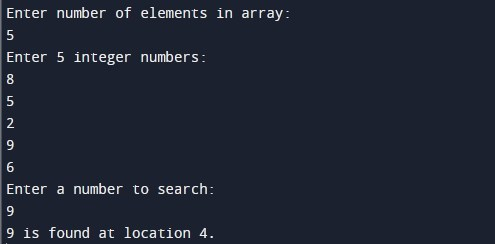# Linear Search Algorithm

In this tutorial you will learn about the Linear Search Algorithm and its application with practical example.

## What Is Linear Search Algorithm?

Linear search algorithm is a simple search algorithm. Linear search algorithm is also known as sequential search algorithm. In this type of search algorithms, we simply search for an element or value in a given array by traversing the all array elements sequentially from the beginning of the array till the desired element or value is found. In Linear search, each array element is matched to find the desired search element. If a match found then location of the matched item is returned else it returns NULL.